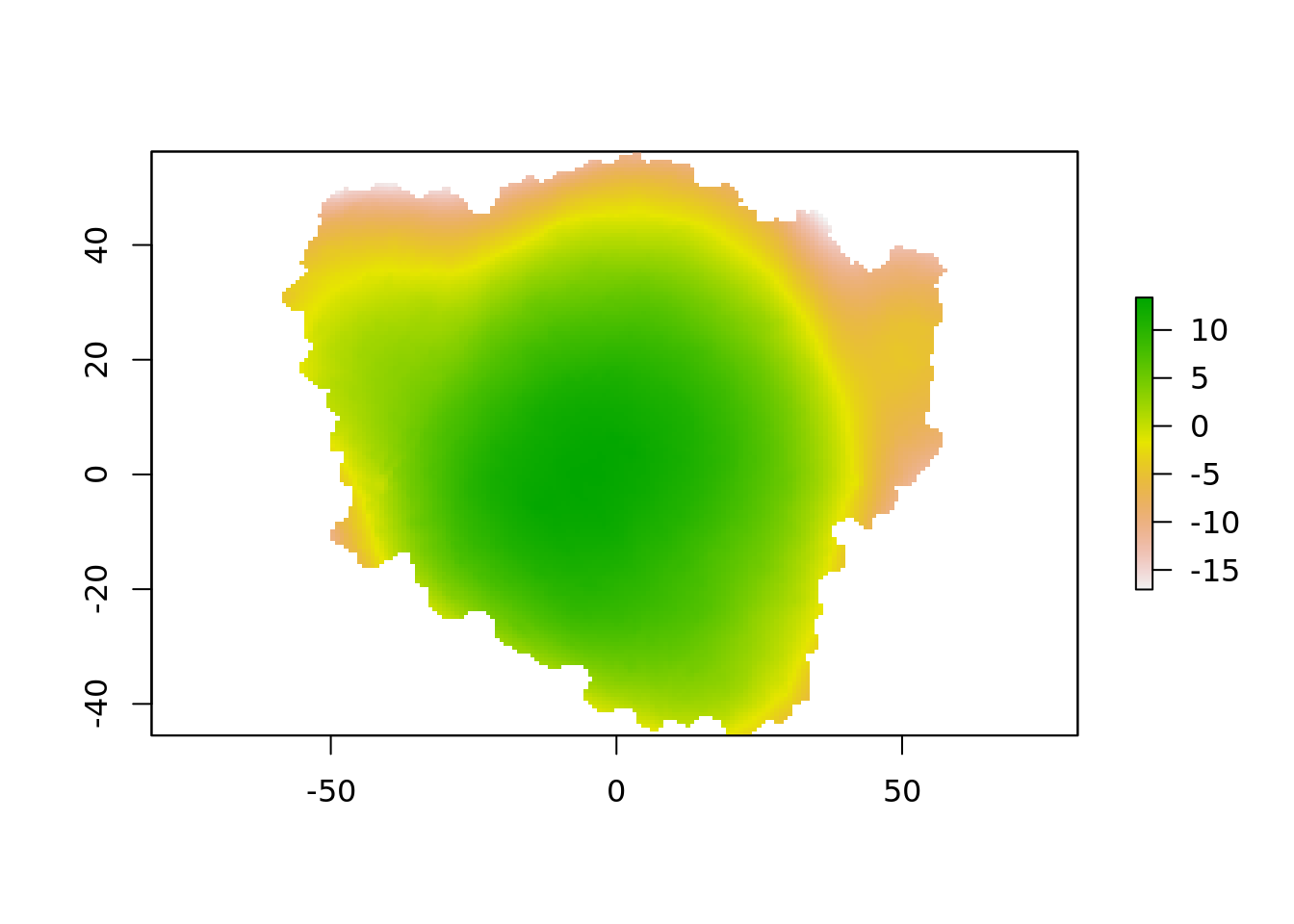#remotes::install_github("marionlouveaux/mgx2r")
filePly <- system.file("extdata", "full/mesh/mesh_meristem_full_T0.ply", package = "mgx2r", mustWork = TRUE)
fileCellGraph <- system.file("extdata",  "full/cellGraph/cellGraph_meristem_full_T0.ply", package = "mgx2r", mustWork = TRUE)
library(mgx2r)
## Warning in rgl.init(initValue, onlyNULL): RGL: unable to open X11 display
## Warning: 'rgl_init' failed, running with rgl.useNULL = TRUE
mgx_palette <- c("#800000", "#FF0000", "#808000", "#FFFF00",
"#008000", "#00FF00", "#008080", "#00FFFF",
"#000080", "#0000FF", "#800080", "#FF00FF")

myMesh <- read_mgxPly(
file = filePly, ShowSpecimen = FALSE, addNormals = TRUE,
MatCol = 1, header_max = 30, my_colors = mgx_palette)
##  "Object has 7763 faces and 4158 vertices."
## Joining, by = "label"
## Joining, by = "parent"
## Joining, by = "cellNr"
## the triangle mesh
tm <- myMesh
library(raster)
## Loading required package: sp
## the xy vertices of the triangles
xy <- t(tm$vb[1:2, ]) ## a target grid grid <- raster(extent(xy), nrows = 150, ncols = 150) rxy <- sp::coordinates(grid) ## the magic barycentric index, the interpolation engine for the target grid vertices ## and where they fall in each triangle relative to triangle vertices pid0 <- geometry::tsearch(xy[,1], xy[,2], t(tm$it), rxy[,1], rxy[, 2],
bary = TRUE)

ok <- !is.na(pid0$idx) r <- setValues(grid, NA_real_) r[ok] <- colSums(matrix(tm$vb[3, tm$it[, pid0$idx[ok]]], nrow = 3) * t(pid0\$p)[, ok])
plot(r)